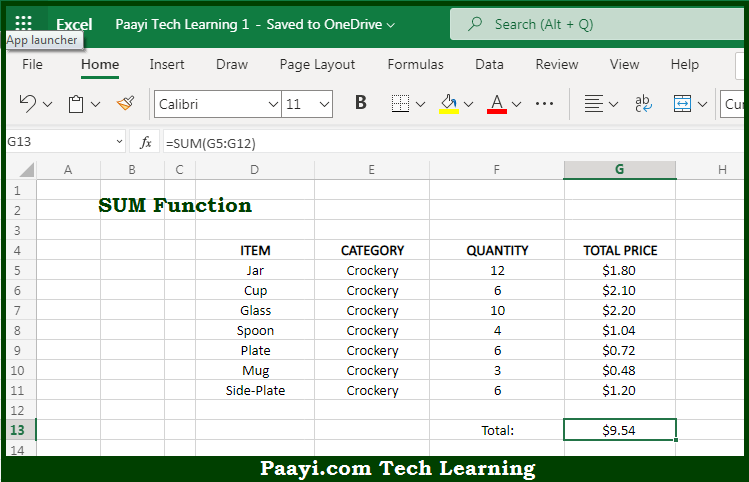# Learn How to Use Microsoft Excel SUM Function

Written by | 0 Comments | 556 Views

In this article, you will learn how to use the Microsoft Excel SUM function and its prime function in Microsoft Excel. You will also get to know the Microsoft Excel SUM function return value and syntax with the help of some examples.

Microsoft Excel SUM Function

The main function of the Microsoft Excel SUM function is to get the sum or total of given numbers. That implies, with the help of the SUM function you can able to return the sum of values supplied. It should be noted that the SUM function can work on values in the form of numbers, cell references, ranges, arrays, and constants, in any combination. SUM function can handle up to 255 individual arguments. So, with the help of the SUM function, you can able to get the subtotal of a given list or database.

Return Value of SUM Function

The return value will be the sum of values supplied.

Syntax of SUM Function

=SUM(numbers1, [number2], [number3], ...)

Where the arguments:

• number1: This is the first value to sum
• number2: This is the second value to sum (optional).
• number3: This is the third value to sum (optional).

## How to Use Microsoft Excel SUM Function?So we know that Microsoft Excel SUM function you can able to get the sum or total of given numbers. That implies, with the help of the SUM function you can able to return a sum of values supplied. It should be noted that the SUM function can work on values in the form of numbers, cell references, ranges, arrays, and constants, in any combination. SUM function can handle up to 255 individual arguments. So, with the help of the SUM function, you can able to get the subtotal of a given list or database.